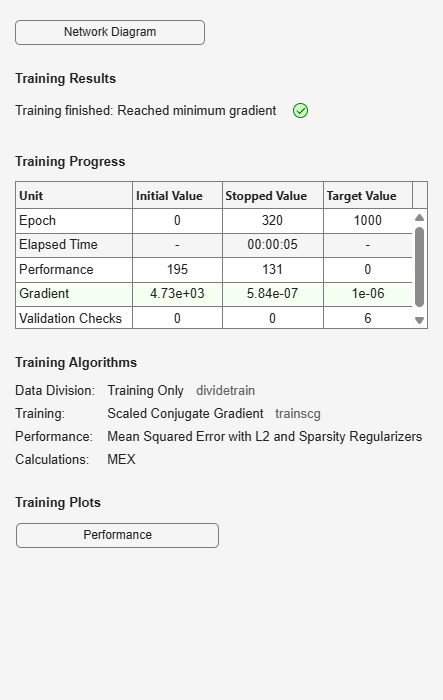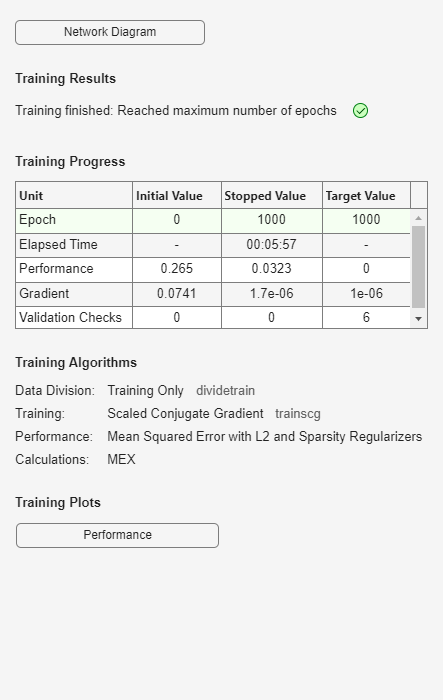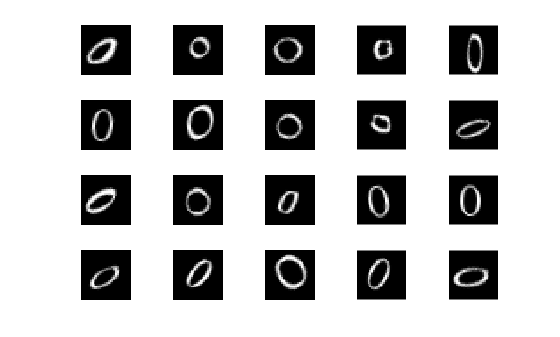# trainAutoencoder

Train an autoencoder

## Syntax

``autoenc = trainAutoencoder(X)``
``autoenc = trainAutoencoder(X,hiddenSize)``
``autoenc = trainAutoencoder(___,Name,Value)``

## Description

example

````autoenc = trainAutoencoder(X)` returns an autoencoder, `autoenc`, trained using the training data in `X`.```
````autoenc = trainAutoencoder(X,hiddenSize)` returns an autoencoder `autoenc`, with the hidden representation size of `hiddenSize`.```

example

````autoenc = trainAutoencoder(___,Name,Value)` returns an autoencoder `autoenc`, for any of the above input arguments with additional options specified by one or more `Name,Value` pair arguments.For example, you can specify the sparsity proportion or the maximum number of training iterations.```

## Examples

collapse all

`X = abalone_dataset;`

`X` is an 8-by-4177 matrix defining eight attributes for 4177 different abalone shells: sex (M, F, and I (for infant)), length, diameter, height, whole weight, shucked weight, viscera weight, shell weight. For more information on the dataset, type `help abalone_dataset` in the command line.

Train a sparse autoencoder with default settings.

`autoenc = trainAutoencoder(X);`

Reconstruct the abalone shell ring data using the trained autoencoder.

`XReconstructed = predict(autoenc,X);`

Compute the mean squared reconstruction error.

`mseError = mse(X-XReconstructed)`
```mseError = 0.0167 ```

`X = abalone_dataset;`

`X` is an 8-by-4177 matrix defining eight attributes for 4177 different abalone shells: sex (M, F, and I (for infant)), length, diameter, height, whole weight, shucked weight, viscera weight, shell weight. For more information on the dataset, type `help abalone_dataset` in the command line.

Train a sparse autoencoder with hidden size 4, 400 maximum epochs, and linear transfer function for the decoder.

```autoenc = trainAutoencoder(X,4,'MaxEpochs',400,... 'DecoderTransferFunction','purelin');```

Reconstruct the abalone shell ring data using the trained autoencoder.

`XReconstructed = predict(autoenc,X);`

Compute the mean squared reconstruction error.

`mseError = mse(X-XReconstructed)`
```mseError = 0.0044 ```

Generate the training data.

```rng(0,'twister'); % For reproducibility n = 1000; r = linspace(-10,10,n)'; x = 1 + r*5e-2 + sin(r)./r + 0.2*randn(n,1);```

Train autoencoder using the training data.

```hiddenSize = 25; autoenc = trainAutoencoder(x',hiddenSize,... 'EncoderTransferFunction','satlin',... 'DecoderTransferFunction','purelin',... 'L2WeightRegularization',0.01,... 'SparsityRegularization',4,... 'SparsityProportion',0.10);```

Generate the test data.

```n = 1000; r = sort(-10 + 20*rand(n,1)); xtest = 1 + r*5e-2 + sin(r)./r + 0.4*randn(n,1);```

Predict the test data using the trained autoencoder, `autoenc` .

`xReconstructed = predict(autoenc,xtest');`

Plot the actual test data and the predictions.

```figure; plot(xtest,'r.'); hold on plot(xReconstructed,'go');``````XTrain = digitTrainCellArrayData; ```

The training data is a 1-by-5000 cell array, where each cell containing a 28-by-28 matrix representing a synthetic image of a handwritten digit.

Train an autoencoder with a hidden layer containing 25 neurons.

```hiddenSize = 25; autoenc = trainAutoencoder(XTrain,hiddenSize,... 'L2WeightRegularization',0.004,... 'SparsityRegularization',4,... 'SparsityProportion',0.15); ```

```XTest = digitTestCellArrayData; ```

The test data is a 1-by-5000 cell array, with each cell containing a 28-by-28 matrix representing a synthetic image of a handwritten digit.

Reconstruct the test image data using the trained autoencoder, `autoenc`.

```xReconstructed = predict(autoenc,XTest); ```

View the actual test data.

```figure; for i = 1:20 subplot(4,5,i); imshow(XTest{i}); end ```View the reconstructed test data.

```figure; for i = 1:20 subplot(4,5,i); imshow(xReconstructed{i}); end ```## Input Arguments

collapse all

Training data, specified as a matrix of training samples or a cell array of image data. If `X` is a matrix, then each column contains a single sample. If `X` is a cell array of image data, then the data in each cell must have the same number of dimensions. The image data can be pixel intensity data for gray images, in which case, each cell contains an m-by-n matrix. Alternatively, the image data can be RGB data, in which case, each cell contains an m-by-n-3 matrix.

Data Types: `single` | `double` | `cell`

Size of hidden representation of the autoencoder, specified as a positive integer value. This number is the number of neurons in the hidden layer.

Data Types: `single` | `double`

### Name-Value Pair Arguments

Specify optional comma-separated pairs of `Name,Value` arguments. `Name` is the argument name and `Value` is the corresponding value. `Name` must appear inside quotes. You can specify several name and value pair arguments in any order as `Name1,Value1,...,NameN,ValueN`.

Example: `'EncoderTransferFunction','satlin','L2WeightRegularization',0.05` specifies the transfer function for the encoder as the positive saturating linear transfer function and the L2 weight regularization as 0.05.

Transfer function for the encoder, specified as the comma-separated pair consisting of `'EncoderTransferFunction'` and one of the following.

Transfer Function OptionDefinition
`'logsig'`

Logistic sigmoid function

`$f\left(z\right)=\frac{1}{1+{e}^{-z}}$`

`'satlin'`

Positive saturating linear transfer function

Example: `'EncoderTransferFunction','satlin'`

Transfer function for the decoder, specified as the comma-separated pair consisting of `'DecoderTransferFunction'` and one of the following.

Transfer Function OptionDefinition
`'logsig'`

Logistic sigmoid function

`$f\left(z\right)=\frac{1}{1+{e}^{-z}}$`

`'satlin'`

Positive saturating linear transfer function

`'purelin'`

Linear transfer function

`$f\left(z\right)=z$`

Example: `'DecoderTransferFunction','purelin'`

Maximum number of training epochs or iterations, specified as the comma-separated pair consisting of `'MaxEpochs'` and a positive integer value.

Example: `'MaxEpochs',1200`

The coefficient for the L2 weight regularizer in the cost function (`LossFunction`), specified as the comma-separated pair consisting of `'L2WeightRegularization'` and a positive scalar value.

Example: `'L2WeightRegularization',0.05`

Loss function to use for training, specified as the comma-separated pair consisting of `'LossFunction'` and `'msesparse'`. It corresponds to the mean squared error function adjusted for training a sparse autoencoder as follows:

where λ is the coefficient for the L2 regularization term and β is the coefficient for the sparsity regularization term. You can specify the values of λ and β by using the `L2WeightRegularization` and `SparsityRegularization` name-value pair arguments, respectively, while training an autoencoder.

Indicator to show the training window, specified as the comma-separated pair consisting of `'ShowProgressWindow'` and either `true` or `false`.

Example: `'ShowProgressWindow',false`

Desired proportion of training examples a neuron reacts to, specified as the comma-separated pair consisting of `'SparsityProportion'` and a positive scalar value. Sparsity proportion is a parameter of the sparsity regularizer. It controls the sparsity of the output from the hidden layer. A low value for SparsityProportion usually leads to each neuron in the hidden layer "specializing" by only giving a high output for a small number of training examples. Hence, a low sparsity proportion encourages higher degree of sparsity. See Sparse Autoencoders.

Example: `'SparsityProportion',0.01` is equivalent to saying that each neuron in the hidden layer should have an average output of 0.1 over the training examples.

Coefficient that controls the impact of the sparsity regularizer in the cost function, specified as the comma-separated pair consisting of `'SparsityRegularization'` and a positive scalar value.

Example: `'SparsityRegularization',1.6`

The algorithm to use for training the autoencoder, specified as the comma-separated pair consisting of `'TrainingAlgorithm'` and `'trainscg'`. It stands for scaled conjugate gradient descent .

Indicator to rescale the input data, specified as the comma-separated pair consisting of `'ScaleData'` and either `true` or `false`.

Autoencoders attempt to replicate their input at their output. For it to be possible, the range of the input data must match the range of the transfer function for the decoder. `trainAutoencoder` automatically scales the training data to this range when training an autoencoder. If the data was scaled while training an autoencoder, the `predict`, `encode`, and `decode` methods also scale the data.

Example: `'ScaleData',false`

Indicator to use GPU for training, specified as the comma-separated pair consisting of `'UseGPU'` and either `true` or `false`.

Example: `'UseGPU',true`

## Output Arguments

collapse all

Trained autoencoder, returned as an `Autoencoder` object. For information on the properties and methods of this object, see `Autoencoder` class page.

collapse all

### Autoencoders

An autoencoder is a neural network which is trained to replicate its input at its output. Autoencoders can be used as tools to learn deep neural networks. Training an autoencoder is unsupervised in the sense that no labeled data is needed. The training process is still based on the optimization of a cost function. The cost function measures the error between the input x and its reconstruction at the output $\stackrel{^}{x}$.

An autoencoder is composed of an encoder and a decoder. The encoder and decoder can have multiple layers, but for simplicity consider that each of them has only one layer.

If the input to an autoencoder is a vector $x\in {ℝ}^{{D}_{x}}$, then the encoder maps the vector x to another vector $z\in {ℝ}^{{D}^{\left(1\right)}}$ as follows:

`$z={h}^{{}^{\left(1\right)}}\left({W}^{\left(1\right)}x+{b}^{\left(1\right)}\right),$`

where the superscript (1) indicates the first layer. ${h}^{\left(1\right)}:{ℝ}^{{D}^{\left(1\right)}}\to {ℝ}^{{D}^{\left(1\right)}}$ is a transfer function for the encoder, ${W}^{\left(1\right)}\in {ℝ}^{{D}^{\left(1\right)}×{D}_{{}^{x}}}$ is a weight matrix, and ${b}^{\left(1\right)}\in {ℝ}^{{D}^{\left(1\right)}}$ is a bias vector. Then, the decoder maps the encoded representation z back into an estimate of the original input vector, x, as follows:

`$\stackrel{^}{x}={h}^{{}^{\left(2\right)}}\left({W}^{\left(2\right)}z+{b}^{\left(2\right)}\right),$`

where the superscript (2) represents the second layer. ${h}^{\left(2\right)}:{ℝ}^{{D}_{x}}\to {ℝ}^{{D}_{x}}$ is the transfer function for the decoder,${W}^{\left(1\right)}\in {ℝ}^{{D}_{{}^{x}}×{D}^{\left(1\right)}}$ is a weight matrix, and ${b}^{\left(2\right)}\in {ℝ}^{{D}_{x}}$ is a bias vector.

### Sparse Autoencoders

Encouraging sparsity of an autoencoder is possible by adding a regularizer to the cost function . This regularizer is a function of the average output activation value of a neuron. The average output activation measure of a neuron i is defined as:

`${\stackrel{^}{\rho }}_{i}=\frac{1}{n}\sum _{j=1}^{n}{z}_{i}^{\left(1\right)}\left({x}_{j}\right)=\frac{1}{n}\sum _{j=1}^{n}h\left({w}_{i}^{\left(1\right)T}{x}_{j}+{b}_{i}^{\left(1\right)}\right),$`

where n is the total number of training examples. xj is the jth training example, ${w}_{i}^{\left(1\right)T}$ is the ith row of the weight matrix ${W}^{\left(1\right)}$, and ${b}_{i}^{\left(1\right)}$ is the ith entry of the bias vector, ${b}^{\left(1\right)}$. A neuron is considered to be ‘firing’, if its output activation value is high. A low output activation value means that the neuron in the hidden layer fires in response to a small number of the training examples. Adding a term to the cost function that constrains the values of ${\stackrel{^}{\rho }}_{i}$ to be low encourages the autoencoder to learn a representation, where each neuron in the hidden layer fires to a small number of training examples. That is, each neuron specializes by responding to some feature that is only present in a small subset of the training examples.

### Sparsity Regularization

Sparsity regularizer attempts to enforce a constraint on the sparsity of the output from the hidden layer. Sparsity can be encouraged by adding a regularization term that takes a large value when the average activation value, ${\stackrel{^}{\rho }}_{i}$, of a neuron i and its desired value, $\rho$, are not close in value . One such sparsity regularization term can be the Kullback-Leibler divergence.

`${\Omega }_{sparsity}=\sum _{i=1}^{{D}^{\left(1\right)}}KL\left(\rho \parallel {\stackrel{^}{\rho }}_{i}\right)=\sum _{i=1}^{{D}^{\left(1\right)}}\rho \mathrm{log}\left(\frac{\rho }{{\stackrel{^}{\rho }}_{i}}\right)+\left(1-\rho \right)\mathrm{log}\left(\frac{1-\rho }{1-{\stackrel{^}{\rho }}_{i}}\right)$`

Kullback-Leibler divergence is a function for measuring how different two distributions are. In this case, it takes the value zero when $\rho$ and ${\stackrel{^}{\rho }}_{i}$ are equal to each other, and becomes larger as they diverge from each other. Minimizing the cost function forces this term to be small, hence $\rho$ and ${\stackrel{^}{\rho }}_{i}$ to be close to each other. You can define the desired value of the average activation value using the `SparsityProportion` name-value pair argument while training an autoencoder.

### L2 Regularization

When training a sparse autoencoder, it is possible to make the sparsity regulariser small by increasing the values of the weights w(l) and decreasing the values of z(1) . Adding a regularization term on the weights to the cost function prevents it from happening. This term is called the L2 regularization term and is defined by:

`${\Omega }_{weights}=\frac{1}{2}{\sum _{l}^{L}\sum _{j}^{n}\sum _{i}^{k}\left({w}_{ji}^{\left(l\right)}\right)}^{2},$`

where L is the number of hidden layers, n is the number of observations (examples), and k is the number of variables in the training data.

### Cost Function

The cost function for training a sparse autoencoder is an adjusted mean squared error function as follows:

where λ is the coefficient for the L2 regularization term and β is the coefficient for the sparsity regularization term. You can specify the values of λ and β by using the `L2WeightRegularization` and `SparsityRegularization` name-value pair arguments, respectively, while training an autoencoder.

 Moller, M. F. “A Scaled Conjugate Gradient Algorithm for Fast Supervised Learning”, Neural Networks, Vol. 6, 1993, pp. 525–533.

 Olshausen, B. A. and D. J. Field. “Sparse Coding with an Overcomplete Basis Set: A Strategy Employed by V1.” Vision Research, Vol.37, 1997, pp.3311–3325.